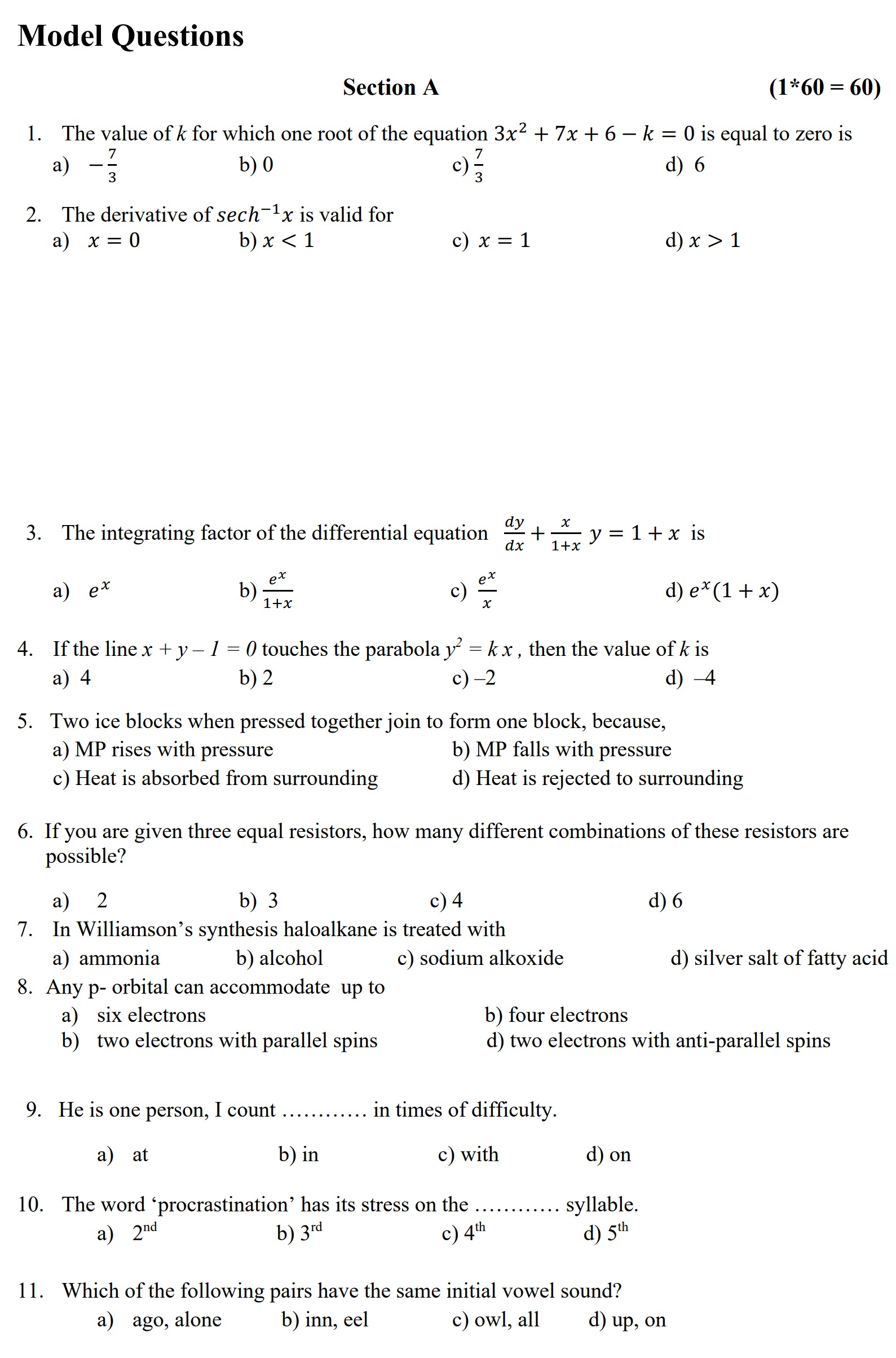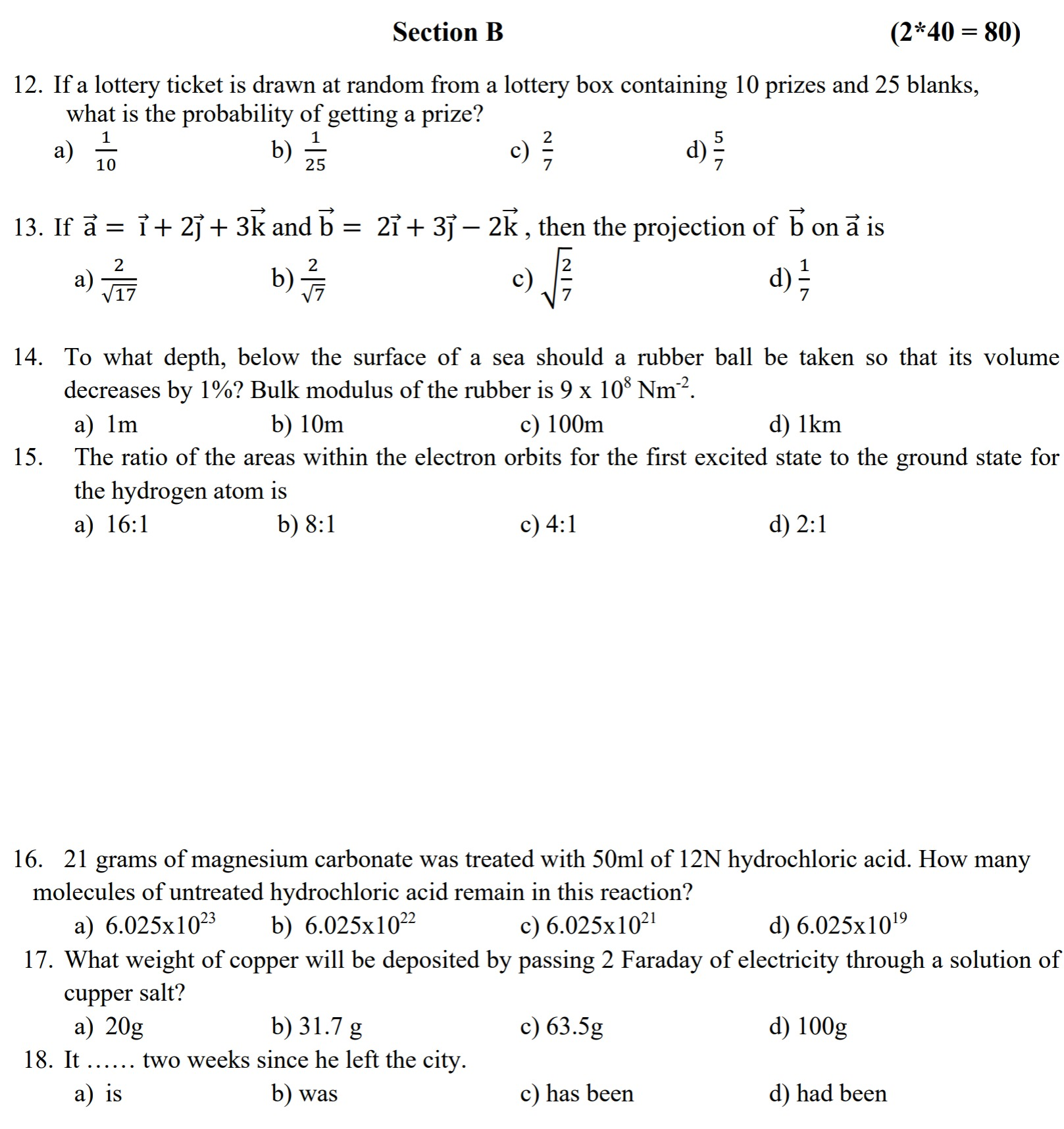# IOE Entrance Model Question 2080 - Latest IOE Model

IOE Entrance Model Question 2080 - Institute of Engineering Provide this Latest model question to prepare for upcoming IOE Entrance Exam 2080/2023.## IOE Entrance Model Question 2080

Institute of Engineering confirmed that IOE Exam 2080 will be start from 25th of Bhandra, 2080. IOE also provide Syllabus and model question to prepare for upcoming IOE Entrance Exam.

IOE just provide sample model question as provided below:

### Section A

1. The value of K for which one root of the equation 3x^2 + 7x + 6 - k = 0 is equal to zero is

a) - \frac{7}{3}

b) 0

c) -\frac{7}{3}

d) 6

2. The derivative of sech^-1x is valid for

a) x=0

b) x<1

c) x=1

d) x>1

3. The integrating factor of the differential equation \frac{dy}{dx}+\frac{x}{1+x}y=1+x is

a) e^x

b) \frac{e^x}{1+x}

c) \frac{e^x}{x}

d) e^x(1+x)

4. If the line x+y-1=0 touches the parabola y^2=kx, the the value of k is

a) 4

b) 2

c) -2

d) -4

5. Two ice blocks when pressed together join to form one block, because,

a) MP rises with pressure

b) MP falls with pressure

c) Heat is absorbed from surrounding

d) Heat is rejected to surrounding

6. If you are given three equal resistors, how many different combinations of these resistors are possible?

a) 2

b) 3

c) 4

d) 6

7. In Williamson’s synthesis haloalkane is treated with

a) ammonia

b) alcohol

c) sodium alkoxide

d) silver salt of fatty acid

8. Any p- orbital can accommodate up to

a) six electrons

b) four electrons

c) two electrons with parallel spins

d) two electrons with anti-parallel spins

9. He is one person, I count ………… in times of difficulty.

a) at

b) in

c) with

d) on

10. The word ‘procrastination’ has its stress on the ………… syllable.

a) 2nd

b) 3rd

c) 4th

d) 5th

11. Which of the following pairs have the same initial vowel sound?

a) ago, alone

b) inn, eel

c) owl, all

d) up, on

### Section B

12. If a lottery ticket is drawn at random from a lottery box containing 10 prizes and 25 blanks, What is the probability of getting a prize?

a) \frac{1}{10}

b) \frac{1}{25}

c) \frac{2}{7}

d) \frac{5}{7}

12. If \underset{a}{\rightarrow } = \underset{i}{\rightarrow } + 2\underset{j}{\rightarrow } + 3\underset{k}{\rightarrow } and \underset{b}{\rightarrow } = 2\underset{i}{\rightarrow } + 3\underset{j}{\rightarrow } - 2\underset{k}{\rightarrow }, then the projection of \underset{b}{\rightarrow } on \underset{a}{\rightarrow } is

a) \frac{2}{\sqrt{17}}

b) \frac{2}{\sqrt{7}}

c) \sqrt{\frac{2}{7}}

d) \frac{1}{7}

14. To what depth, below the surface of a sea should a rubber ball be taken so that its volume decreases by 1%? Bulk modulus of the rubber is 9 x 10^8 Nm^-2.

a) 1m

b) 10m

c) 100m

d) 1km

15. The ratio of the areas within the electron orbits for the first excited state to the ground state for the hydrogen atom is

a) 16:1

b) 8:1

c) 4:1

d) 2:1

16. 21 grams of magnesium carbonate was treated with 50ml of 12N hydrochloric acid. How many molecules of untreated hydrochloric acid remain in this reaction?

a) 6.025 * 10^23

b) 6.025 * 10^22

c) 6.025 * 10^21

d) 6.025 * 10^19

17. What weight of copper will be deposited by passing 2 Faraday of electricity through a solution of cupper salt?

a) 20g

b) 31.7g

c) 63.5g

d) 100g

18. It …… two weeks since he left the city.

a) is

b) was

c) has been

19. Read the following passage carefully and answer the questions given below by selecting the
correct option.

Language, as they say, is the lens through which human beings perceive the world. If so, English is
perhaps the most distorting lens through which to see animals. It has perpetuated a cross-eyed view of
birds, beasts, fish and fowl. The very word ‘animal’ connotes the brutish and the sensual. Animal
instinct implies baseness and vulgarity. The language transfers negative human traits to animals,
making the former appear as characteristics of the latter. Thus, the chicken is cowardly, frightened,
faint-hearted; the goat lustful and foolish; the bear rough and ill-bred. Butterflies are flighty, seals
slippery and foxes notorious for craftiness and cunning. Much the worst are the reptiles particularly the
snake, creeping, base, malignant, abject, ungrateful and treacherous. Always the snake in the grass.
Each species carries its denigration forever embedded in its English name giving the language as many
unpleasant adjectives as it could possibly want. To be bull-headed is to be impetuous and obstinate; the
cattish woman is spiteful and backbiting. An elephant’s walk is ungainly, bird-brains are to be ridiculed
and the herd mentality draws only contempt. You can be as blind as a bat, and batty if you are crazy as
well.
In the English language, the animals are
• a) used for uninteresting comparisons
• b) used for unpleasant comparisons
• c) used for pleasant comparisons
• d) not used for any comparisons

### IOE Model Question 2080 (Images)## IOE Entrance Model Question PDF

You can check this pdf of IOE Entrance Exam to better understand, IOE Question Pattern:

Check:

Getting Info...